Instead of using exact numbers, we often use rough numbers. It is called approximate numbers, and we often use rough numbers in our daily lives.

A concept that is used in rough numbers is rounding. By rounding off a number, we use a rough number.

Why do we need to use rough numbers instead of exact numbers? Also, how can we round off numbers in elementary school math? We will explain how to round.

## Why We Use Approximate Numbers in Everyday Life

In arithmetic, we use approximate numbers. Why do we use approximate numbers? Because there are many situations where it is more convenient to use approximate numbers.

For example, what is the population of the city you live in? The population varies depending on the city you live in; 300,000, 1,000,000, etc. Each city has its own population.

So why don’t we use an exact number for population, such as 1,012,948? It seems to be better to use an exact number. However, it is not possible to know the exact population. The reason is that the population varies from day to day as people move from one place to another.

However, even if we don’t know the exact population, we can know the approximate population. This is why we use approximate population instead of exact numbers.

In addition, we often use approximate numbers to make the discussion easier to understand. For example, rather than being told that the price of a car is \$13,219.53, it is easier to be told that a car costs about \$13,200. By ignoring the lower digits, it is easier to understand the price.

Thus, rough numbers are used in many situations. So, we need to learn rounding in math.

### Rounding Down or Up Numbers by Rounding

How do we round numbers? Rounding is the process of making numbers to zero. To be more precise, it simplifies numbers.

In rounding, the following numbers are reduced to zero.

• 0, 1, 2, 3, 4

For example, suppose the number of participants in an event is 51,240. In this case, it is often said that the number of participants is 50,000. This is because the numbers have been rounded off as follows.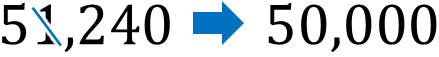For numbers between 0 and 4, rounding results in 0, which is an approximate number. This is called rounding down. This kind of rounding is often used in newspapers and TV news.

On the other hand, what should we do when the number to be rounded is between 5 and 9? In this case, change the number to one higher.

For example, suppose the number of participants in an event is 58,493. In this case, we can say that the number of participants is 60,000. You can simplify the number by rounding it off as follows.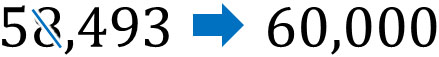If the number is between 5 and 9, increase the number to the left by one. In rounding, this is called rounding up.

When rounding, distinguish whether the target number is 0-4 or 5-9. Whether to round down or up depends on the target number.

## How to Do Actual Rounding?

How do you actually round a number? It is up to you to decide which part of the number you want to round off.

For example, the number 58,493 has a different approximate number depending on where you round it, as shown below.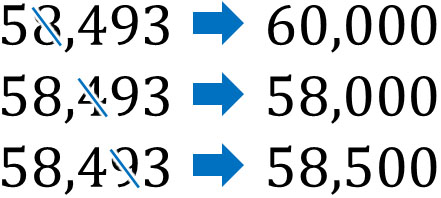Check in advance which numbers you want to round off.

### Rounding to the Nearest A

In math problems, you will be specified where to round. So, we need to understand where to round.

When rounding, you may be asked to round a number to the nearest A. For example, you may be asked like this.

• Round 24,685 to the nearest 100.

The meaning of this is to round the tens place. Therefore, the answer is as follows.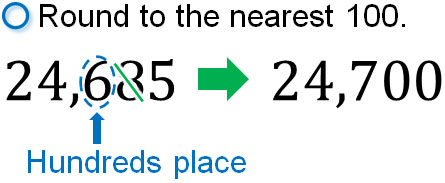In short, focus on the one lower place. For reference, rounding off 24685 gives the following.

• Round 24,685 to the nearest 10,000: 20,000.
• Round 24,685 to the nearest 1,000: 25000.
• Round 24,685 to the nearest 100: 24700.
• Round 24,685 to the nearest 10: 24690.

When rounding to a rough number, check which place you need to round.

### Rounding to A Significant Figures

When rounding, instead of focusing on the place, we sometimes focus on the number of digits from the left—for example, rounding the third digit from the left. For each digit, we count from left to right.

In this case, it is expressed as rounding to A significant figures. For example, it looks like this.

• Round 54,827 to 2 significant figures.

What this means is that we need to round off the third place from the left. So let’s round off the third number from the left as follows.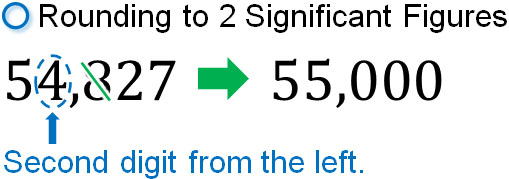Thus, let’s focus on the number of digits one lower. You can get the answer by checking the number one digit lower and rounding it off.

For reference, if we round off 54,827, we get the following.

• 54,827 rounded to 1 significant digit is 50000.
• 54,827 rounded to 2 significant digits is 55000.
• 54,827 rounded to 3 significant digits is 54800.
• 54,827 rounded to 4 significant digits is 54830.

Let’s round off the numbers in this way.

### Rounding Numbers with a Decimal Point

On the other hand, there are cases that you need to round decimals. How do we round numbers that have a decimal point?

If a number has a decimal point, be sure to round it to the correct place. For example, how would you round the following problem?

• Round 2.4735 to tenths.

In this case, you need to round the number two places right from the decimal point. This means leaving one number to the right from the decimal points. It will look like this.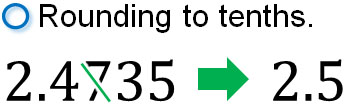For reference, rounding off 2.4735 gives the following.

• 2.4735 rounded to ones is 2.
• 2.4735 rounded to tenths is 2.5.
• 2.4735 rounded to hundredths is 2.47.
• 2.4735 rounded to thousandths is 2.474.

In this way, we round decimals.

## Rounding Numbers to Make Approximate Numbers

Approximate numbers are used frequently in our daily lives. In newspapers and TV news, most numbers are presented as approximate numbers. There is no point in giving exact numbers, and rough numbers are easier for us to understand.

So, let’s understand the meaning of rounding. We also need to learn how to round.

When solving math problems, check which part you need to round off. By rounding to the correct place, you can get the answer.